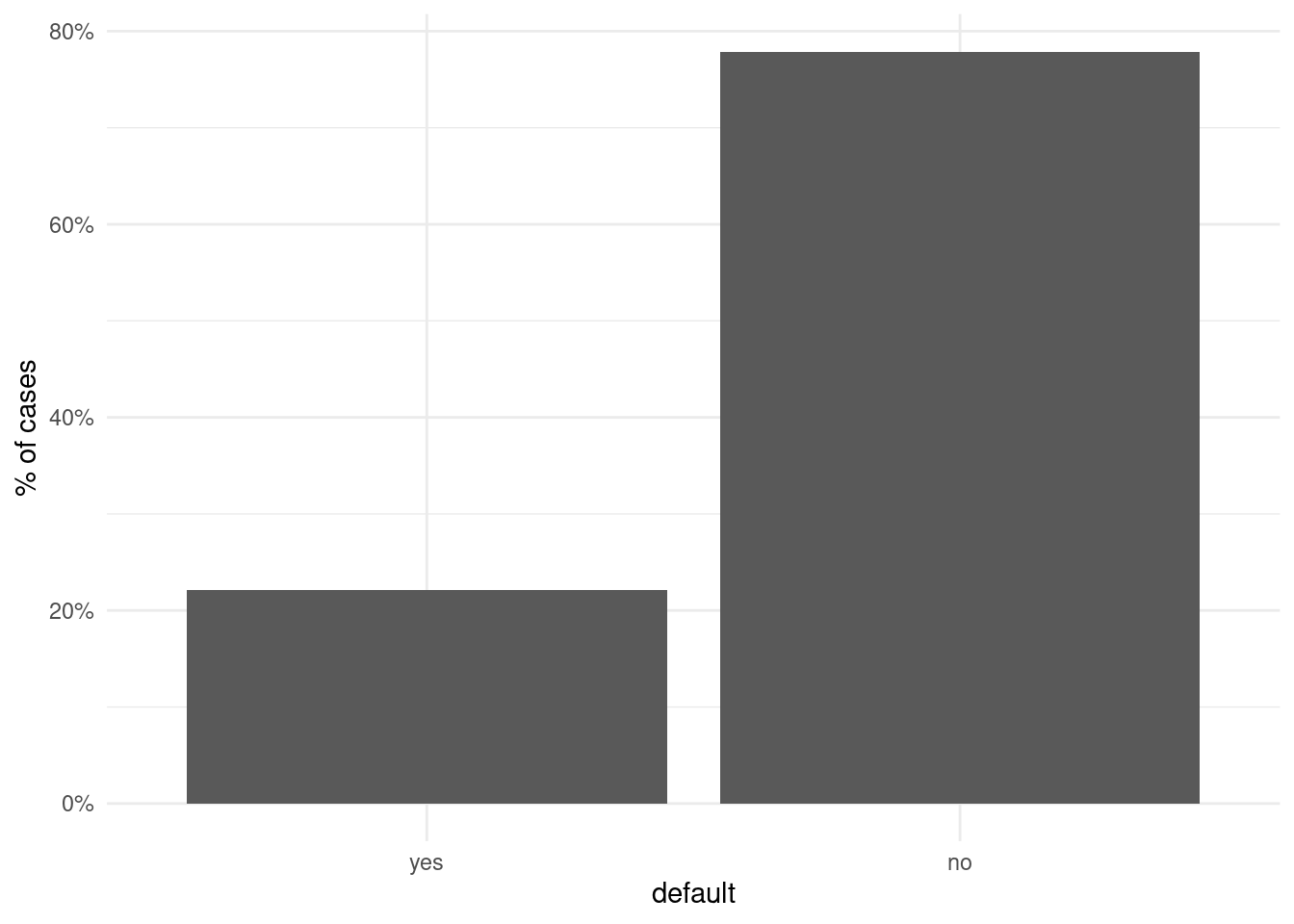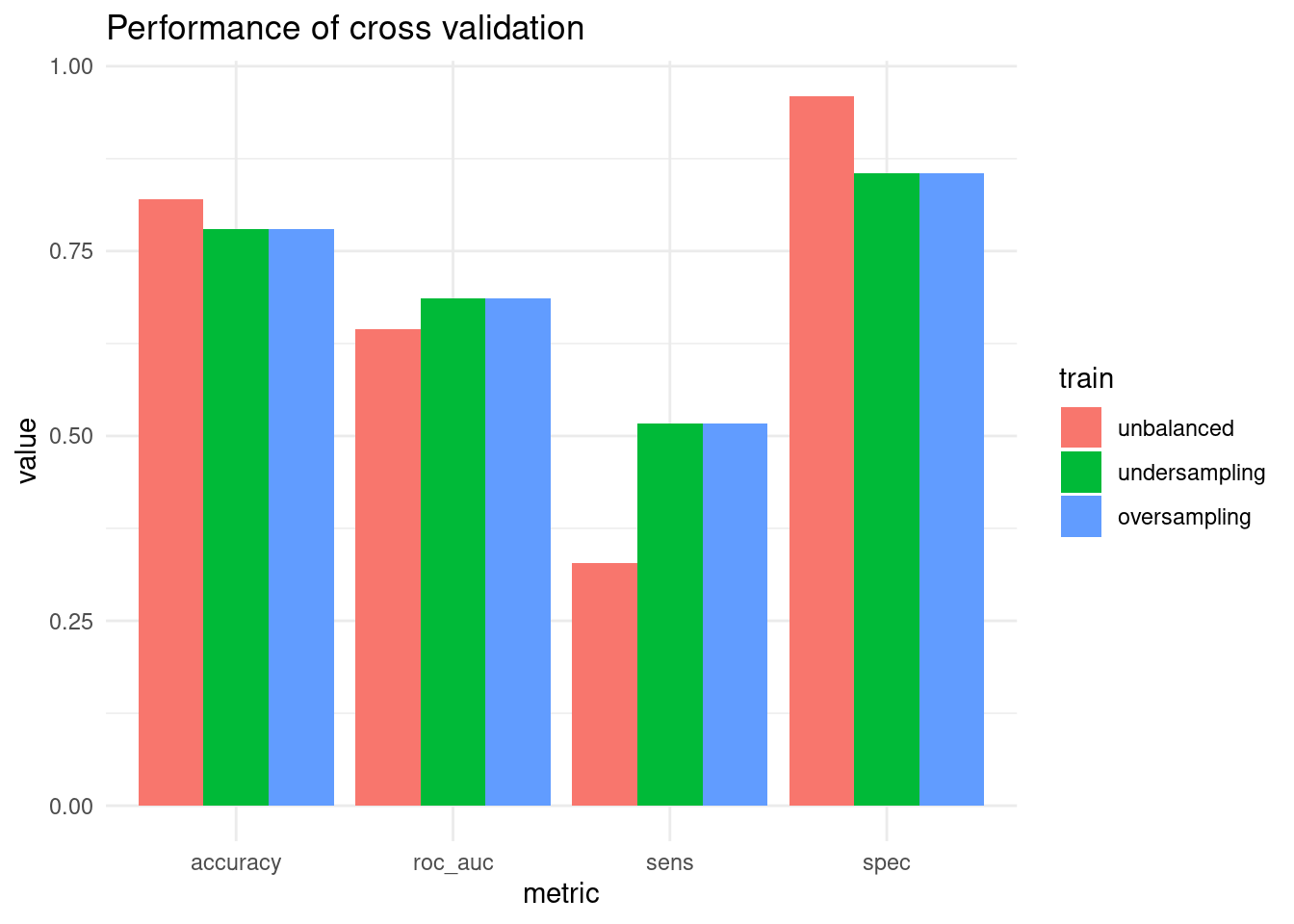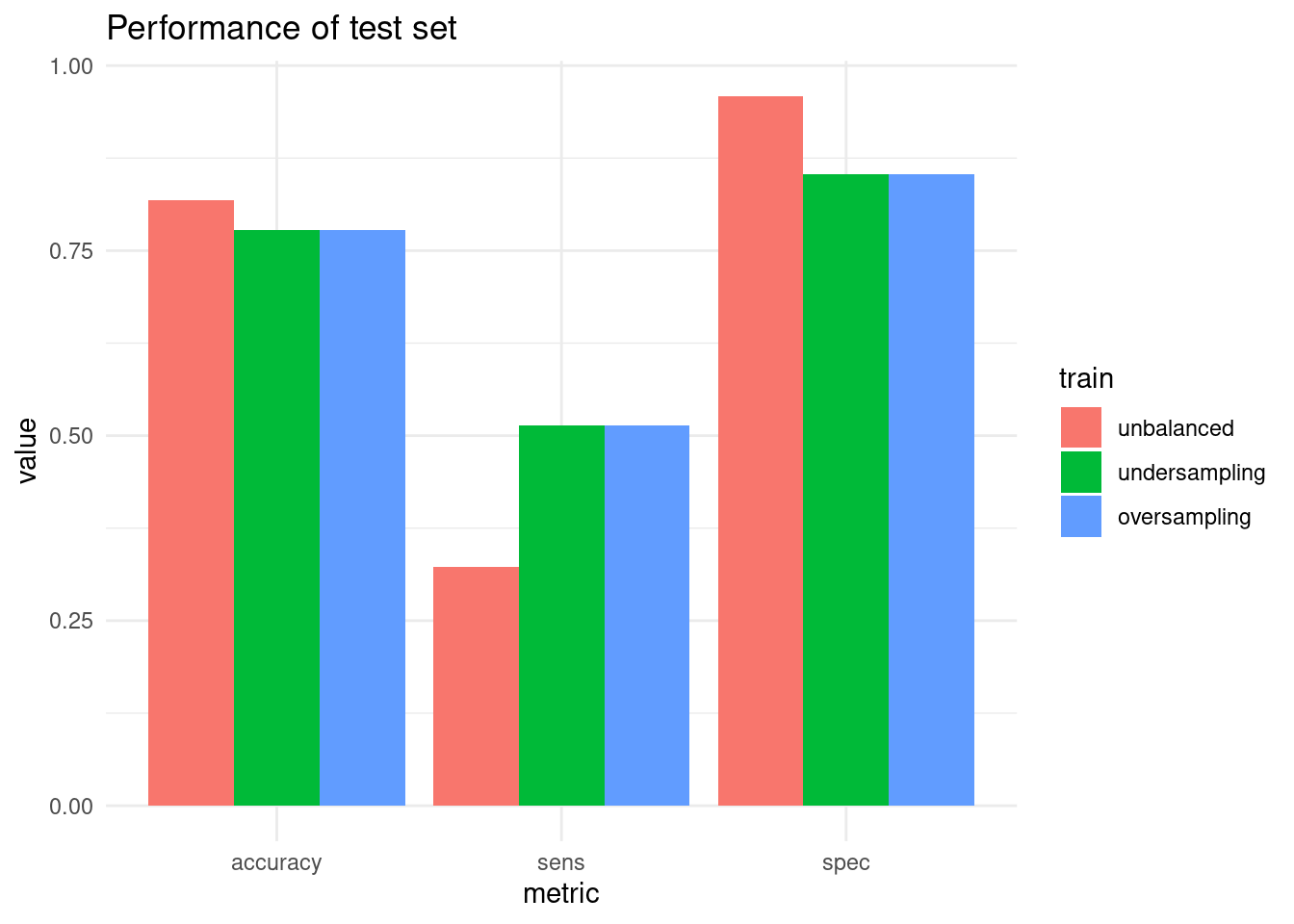# Undersampling and oversampling with tidymodels

In this post, I will present how oversampling and undersampling can help us in a classification job on an unbalanced dataset. In unbalanced datasets, the target variable has an uneven distribution, with salient majority and minority classes. Undersampling and oversampling try to improve model performance training the model with a balanced dataset. When using undersampling, we train the model with a set removing observations of the majority class. With **oversampling*, we train the model with a dataset with additional artificial elements of the minority class.

I will be using `tidymodels` for the prediction workflow, `BAdatasets` to access the dataset and `themis` to perform undersampling and oversampling.

``````library(tidymodels)
library(themis)

I will be using the `LoanDefaults` dataset. It is a dataset of defaults payments in Taiwan, presented in Yeh & Lien (2009).

``data("LoanDefaults")``

When plotting the proportion of observations for each case, we observe that the number of negative cases (not defaulted) is much larger than the positive cases (defaulted). Therefore, the set of positive cases is the minority class. This is a frequent situation in contexts like loan defaults or credit card fraud.

``````LoanDefaults %>%
ggplot(aes(factor(default))) +
geom_bar(aes(y = (..count..)/sum(..count..))) +
scale_y_continuous(labels=percent) +
labs(x = "default", y = "% of cases") +
theme_minimal()``````Let’s define the elements of the `tidymodels` workflow. We start transforming the target `default` variable into a factor, setting the level of positive cases first.

``````LoanDefaults <- LoanDefaults %>%
mutate(default = factor(default, levels = c("1", "0")))``````

Then, we need to split the data into train and test. As the dataset is large, I have selected a large `prop` value. Being the dataset unbalanced, it is important to set `strata = default`, so that the distribution of target variable in train and test sets will be similar to the original dataset. I have applied a similar logic to split the training set into five `folds` to apply cross validation.

``````set.seed(1313)
split <- initial_split(LoanDefaults, prop = 0.9, strata = "default")

folds <- vfold_cv(training(split), v = 5, strata = "default")``````

To evaluate model performance, I have chosen the following metrics:

• `accuracy`: fraction of observations correctly classified.
• sensitivity `sens`: the fraction of positive elements correctly classified.
• specificity `spec`: the fraction of negative elements correctly classified.
• area under the ROC curve `roc_auc`: a parameter assessing the tradeoff between `sens` and `spec`.
``class_metrics <- metric_set(accuracy, sens, spec, roc_auc)``

In unbalanced datasets, accuracy can be a bad metric of performance. If 90% of observations, we obtain an accuracy of 0.9 simply classifying all observations as negative. In that context, usually sensitivity is a more adequate metric. Additionnally, in jobs like loan defaults or credit card fraud, the cost of a false negative is much higher than of a false positive.

I will use a decision tree `dt` model, setting standard parameters.

``````dt <- decision_tree(mode = "classification", cost_complexity = 0.1, min_n = 10) %>%
set_engine("rpart")``````

The preprocessing recipe has the following steps:

• variables `ID` and `PAY0` to `PAY6` are removed. Before that, I am replacing `PAY_0` with payment_status.
• variables `payment_status`, `MARRIAGE` and `EDUCATION` are transformed so that abormal values are set to `NA`.
• `NA` values of predictors are imputed usign k nearest neighbors.
• `SEX`, `MARRIAGE` and `EDUCATION` are transformed into factors.
``````rec_base <- training(split) %>%
recipe(default ~ .) %>%
step_rm(ID) %>%
step_mutate(payment_status = ifelse(PAY_0 < 1, 0, PAY_0)) %>%
step_rm(PAY_0:PAY_6) %>%
step_mutate(MARRIAGE = ifelse(!MARRIAGE %in% 1:3, NA, MARRIAGE)) %>%
step_mutate(EDUCATION = ifelse(!EDUCATION %in% 1:4, NA, EDUCATION)) %>%
step_impute_knn(all_predictors(), neighbors = 3) %>%
step_num2factor(SEX, levels = c("male", "female")) %>%
step_num2factor(MARRIAGE, levels = c("marriage", "single", "others")) %>%
step_num2factor(EDUCATION, levels = c("graduate", "university", "high_school", "others"))``````

The steps to perform undersampling and oversampling are provided by `themis` package. Here I am using the following methods:

• `step_downsample` performs random majority under-sampling with replacement.
• `step_upsample` performs random minority over-sampling with replacement.

These steps are added to the `rec_base` recipe to obtain new recipes `rec_us` and `rec_os` for under and oversampling, respectively.

``````rec_us <- rec_base %>%
step_downsample(default, under_ratio = 1)

rec_os <- rec_base %>%
step_upsample(default, over_ratio = 1)``````

## Testing under- and oversampling with cross validation

Now we are ready to test each of the three models with cross validation. ´cv_unb`,`cv_us`and`cv_os` train the model with the original dataset, undersampling and oversampling respectively.

``````cv_unb <- fit_resamples(object = dt,
preprocessor = rec_base,
resamples = folds,
metrics = class_metrics)

cv_us <- fit_resamples(object = dt,
preprocessor = rec_us,
resamples = folds,
metrics = class_metrics)

cv_os <- fit_resamples(object = dt,
preprocessor = rec_os,
resamples = folds,
metrics = class_metrics)``````

Now we can extract the metrics for each of the cross-validations. We can stack them all in a single data frame `m`.

``````m_unb <- collect_metrics(cv_unb) %>%
mutate(train = "unbalanced")

m_us <- collect_metrics(cv_us) %>%
mutate(train = "undersampling")

m_os <- collect_metrics(cv_os) %>%
mutate(train = "oversampling")

m <- bind_rows(m_unb, m_us, m_os) %>%
mutate(train = factor(train, levels = c("unbalanced", "undersampling", "oversampling")))``````

Let’s see the results:

``````ggplot(m, aes(.metric, mean, fill = train)) +
geom_bar(stat = "identity", position = "dodge") +
theme_minimal() +
labs(title = "Performance of cross validation", x = "metric", y = "value")``````In this case, oversampling and undersampling obtain similar results. Both allow improving sensitivity significantly (although the obtained value is quite poor), paying the price of worsening specificity. Global parameters like accuracy and AUC are not signficantly affected.

## Evaluating the model in the test set

Now I will fit the model on the train set and examine its performance in the test set. In `class_metrics2` I am excluding `roc_auc` because I will obtain the class predicted only.

``class_metrics2 <- metric_set(accuracy, sens, spec)``

Objects `unb_model`, `us_model` and `os_model` contain the model trained in the original, undersampled and oversampled recipes respectively.

``````unb_model <- workflow() %>%
fit(training(split))

us_model <- workflow() %>%
fit(training(split))

os_model <- workflow() %>%
fit(training(split))``````

Next, I am storing in `df_test` the results of evaluating each of the models in the test set. Note that we evaluate the model in the original dataset. Under- and oversampling are only performed to train the model. The datasets where the model is evaluated are not modified.

``````m_test <- lapply(list(unb_model, us_model, os_model), function(x) x %>%
predict(testing(split)) %>%
bind_cols(testing(split)) %>%
class_metrics2(truth = default, estimate = .pred_class))
df_test <- bind_rows(m_test) %>%
mutate(train = rep(c("unbalanced", "undersampling", "oversampling"), each = 3)) %>%
mutate(train = factor(train, levels = c("unbalanced", "undersampling", "oversampling")))``````

Here are the results of the evaluation. They are quite similar to the obtained with cross validation.

``````ggplot(df_test, aes(.metric, .estimate, fill = train)) +
geom_bar(stat = "identity", position = "dodge") +
theme_minimal() +
labs(title = "Performance of test set", x = "metric", y = "value")``````Under- and oversampling can be useful techniques to improve the ratio of true evaluations of the minority class in unbalanced datasets. So these techniques tend to increase sensitivity at the price of worse values of specificity. This can be a good compromise in classification jobs where the cost of a false negative is much higher than of a false positive.

## Session info

``````## R version 4.2.1 (2022-06-23)
## Platform: x86_64-pc-linux-gnu (64-bit)
## Running under: Linux Mint 19.2
##
## Matrix products: default
## BLAS:   /usr/lib/x86_64-linux-gnu/openblas/libblas.so.3
## LAPACK: /usr/lib/x86_64-linux-gnu/libopenblasp-r0.2.20.so
##
## locale:
##   LC_CTYPE=es_ES.UTF-8       LC_NUMERIC=C
##   LC_TIME=es_ES.UTF-8        LC_COLLATE=es_ES.UTF-8
##   LC_MONETARY=es_ES.UTF-8    LC_MESSAGES=es_ES.UTF-8
##   LC_PAPER=es_ES.UTF-8       LC_NAME=C
##  LC_MEASUREMENT=es_ES.UTF-8 LC_IDENTIFICATION=C
##
## attached base packages:
##  stats     graphics  grDevices utils     datasets  methods   base
##
## other attached packages:
##   rpart_4.1.16       BAdatasets_0.1.0   themis_0.2.1       yardstick_0.0.9
##   workflowsets_0.2.1 workflows_0.2.6    tune_0.2.0         tidyr_1.2.0
##   tibble_3.1.6       rsample_0.1.1      recipes_0.2.0      purrr_0.3.4
##  parsnip_0.2.1      modeldata_0.1.1    infer_1.0.0        ggplot2_3.3.5
##  dplyr_1.0.9        dials_0.1.1        scales_1.2.0       broom_0.8.0
##  tidymodels_0.2.0
##
## loaded via a namespace (and not attached):
##   lubridate_1.8.0    doParallel_1.0.17  DiceDesign_1.9     tools_4.2.1
##   backports_1.4.1    bslib_0.3.1        utf8_1.2.2         R6_2.5.1
##   DBI_1.1.2          colorspace_2.0-3   nnet_7.3-17        withr_2.5.0
##  tidyselect_1.1.2   parallelMap_1.5.1  compiler_4.2.1     cli_3.3.0
##  labeling_0.4.2     bookdown_0.26      sass_0.4.1         checkmate_2.1.0
##  stringr_1.4.0      digest_0.6.29      rmarkdown_2.14     unbalanced_2.0
##  pkgconfig_2.0.3    htmltools_0.5.2    parallelly_1.31.1  lhs_1.1.5
##  highr_0.9          fastmap_1.1.0      rlang_1.0.2        rstudioapi_0.13
##  BBmisc_1.12        FNN_1.1.3          farver_2.1.0       jquerylib_0.1.4
##  generics_0.1.2     jsonlite_1.8.0     magrittr_2.0.3     ROSE_0.0-4
##  Matrix_1.5-1       Rcpp_1.0.8.3       munsell_0.5.0      fansi_1.0.3
##  GPfit_1.0-8        lifecycle_1.0.1    furrr_0.3.0        stringi_1.7.6
##  pROC_1.18.0        yaml_2.3.5         MASS_7.3-58        plyr_1.8.7
##  grid_4.2.1         parallel_4.2.1     listenv_0.8.0      crayon_1.5.1
##  lattice_0.20-45    splines_4.2.1      mlr_2.19.0         knitr_1.39
##  pillar_1.7.0       future.apply_1.9.0 codetools_0.2-18   fastmatch_1.1-3
##  glue_1.6.2         evaluate_0.15      ParamHelpers_1.14  blogdown_1.9
##  data.table_1.14.2  vctrs_0.4.1        foreach_1.5.2      RANN_2.6.1
##  gtable_0.3.0       future_1.25.0      assertthat_0.2.1   xfun_0.30
##  gower_1.0.0        prodlim_2019.11.13 class_7.3-20       survival_3.4-0
##  timeDate_3043.102  iterators_1.0.14   hardhat_0.2.0      lava_1.6.10
##  globals_0.14.0     ellipsis_0.3.2     ipred_0.9-12``````